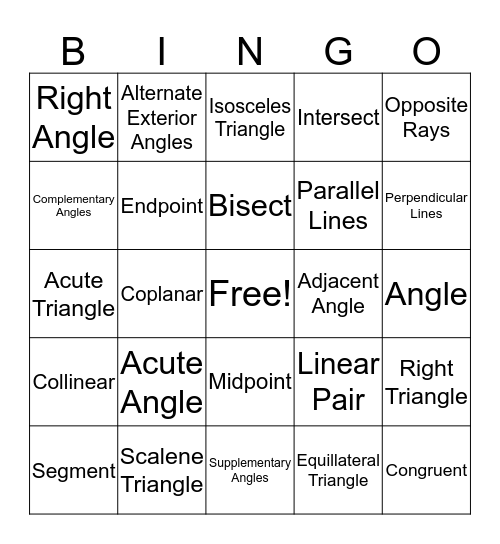# Geometry BingoThis bingo card has a free space and 39 words: Point, Line, Plane, Endpoint, Segment, Midpoint, Bisect, Ray, Opposite Rays, Collinear, Coplanar, Intersect, Congruent, Angle, Adjacent Angle, Acute Angle, Obtuse Angle, Right Angle, Straight Angle, Linear Pair, Complementary Angles, Supplementary Angles, Parallel Lines, Perpendicular Lines, Skew Lines, Parallel Planes, Transversal, Corresponding Angles, Alternate Interior Angles, Alternate Exterior Angles, Same-side Interior Angles, Vertical angles, Isosceles Triangle, Scalene Triangle, Equillateral Triangle, Equiangular Triangle, Right Triangle, Acute Triangle and Obtuse Triangle.

## Play Online